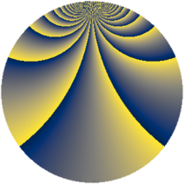# Properties

 Label 276.2.oLevel $276$ Weight $2$ Character orbit 276.o Rep. character $\chi_{276}(35,\cdot)$ Character field $\Q(\zeta_{22})$ Dimension $440$ Newform subspaces $1$ Sturm bound $96$ Trace bound $0$

# Related objects

## Defining parameters

 Level: $$N$$ $$=$$ $$276 = 2^{2} \cdot 3 \cdot 23$$ Weight: $$k$$ $$=$$ $$2$$ Character orbit: $$[\chi]$$ $$=$$ 276.o (of order $$22$$ and degree $$10$$) Character conductor: $$\operatorname{cond}(\chi)$$ $$=$$ $$276$$ Character field: $$\Q(\zeta_{22})$$ Newform subspaces: $$1$$ Sturm bound: $$96$$ Trace bound: $$0$$

## Dimensions

The following table gives the dimensions of various subspaces of $$M_{2}(276, [\chi])$$.

Total New Old
Modular forms 520 520 0
Cusp forms 440 440 0
Eisenstein series 80 80 0

## Trace form

 $$440q - 22q^{4} - 8q^{6} - 18q^{9} + O(q^{10})$$ $$440q - 22q^{4} - 8q^{6} - 18q^{9} - 30q^{10} - 18q^{12} - 36q^{13} - 30q^{16} - 10q^{18} - 22q^{21} - 48q^{22} - 14q^{24} - 8q^{25} - 34q^{28} - 21q^{30} - 6q^{33} - 32q^{34} + 22q^{36} - 36q^{37} - 168q^{40} + q^{42} - 44q^{45} - 110q^{46} - 6q^{48} - 16q^{49} - 94q^{52} + 21q^{54} - 46q^{57} - 116q^{58} - 47q^{60} - 68q^{61} - 28q^{64} - 20q^{66} - 22q^{69} - 44q^{70} - 23q^{72} - 36q^{73} - 74q^{76} + 134q^{78} - 130q^{81} + 36q^{82} + 105q^{84} - 60q^{85} + 38q^{88} + 162q^{90} - 332q^{93} - 40q^{94} + 225q^{96} - 36q^{97} + O(q^{100})$$

## Decomposition of $$S_{2}^{\mathrm{new}}(276, [\chi])$$ into newform subspaces

Label Dim. $$A$$ Field CM Traces $q$-expansion
$$a_2$$ $$a_3$$ $$a_5$$ $$a_7$$
276.2.o.a $$440$$ $$2.204$$ None $$0$$ $$0$$ $$0$$ $$0$$